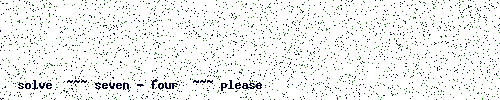## 2021-08-10

### Extra mode for the pan and fade modules

Added an extra mode (lin2) to the Pan, XFade and XFadeStereo modules. It just has twice the output amplitude as the Linear mode. I’ve added it as the last mode to keep older patches compatible.

Published as pre-release. 2021.8.10.0

StereoPan has no modes at all currently, it works a bit differently:

```      PPosition  :=        FParams[ p_position ];
PPosModAmt :=        FParams[ p_posmodamt];
InType     := Round( FParams[ p_intype  ]);

for zz := 0 to Polyphony - 1
do begin
Control := 1.0 + Clip( PPosition + SignalForInputType( FInputs[ i_control][ zz], InType) * PPosModAmt, -1.0, 1.0);
In1     := FInputs[ i_in1][ zz];
In2     := FInputs[ i_in2][ zz];
Sum     := In1 + In2;
A1      := Bounce( Control, False);
A2      := Clip  ( 1.0 - Control, 0.0, 1.0);
A3      := Clip  ( Control - 1.0, 0.0, 1.0);
A4      := Bounce( Control , False);
FOutputs[ o_out1][ zz] := A1 * In1 + A2 * Sum;
FOutputs[ o_out2][ zz] := A3 * Sum + A4 * In2;
end;
```

Where Bounce is a sort of a wrapping clip … and it does :

```  function  Bounce( const aValue: TSignal; AbsVal: Boolean = False): TSignal; inline;
begin
Result := aValue;

if AbsVal
then begin
if   Result < 0.0
then Result :=       - Result;

if Result > 1.0
then Result :=   2.0 - Result;
end
else begin
if   Result < - 1.0
then Result := - 2.0 - Result
else if Result > 1.0
then Result :=   2.0 - Result;
end;
end;
```

and please solve the "anti bot puzzle", when it is unreadable or otherwise unresolvable you can refresh the page (F5)

the answer must be entered as a number (in digits)